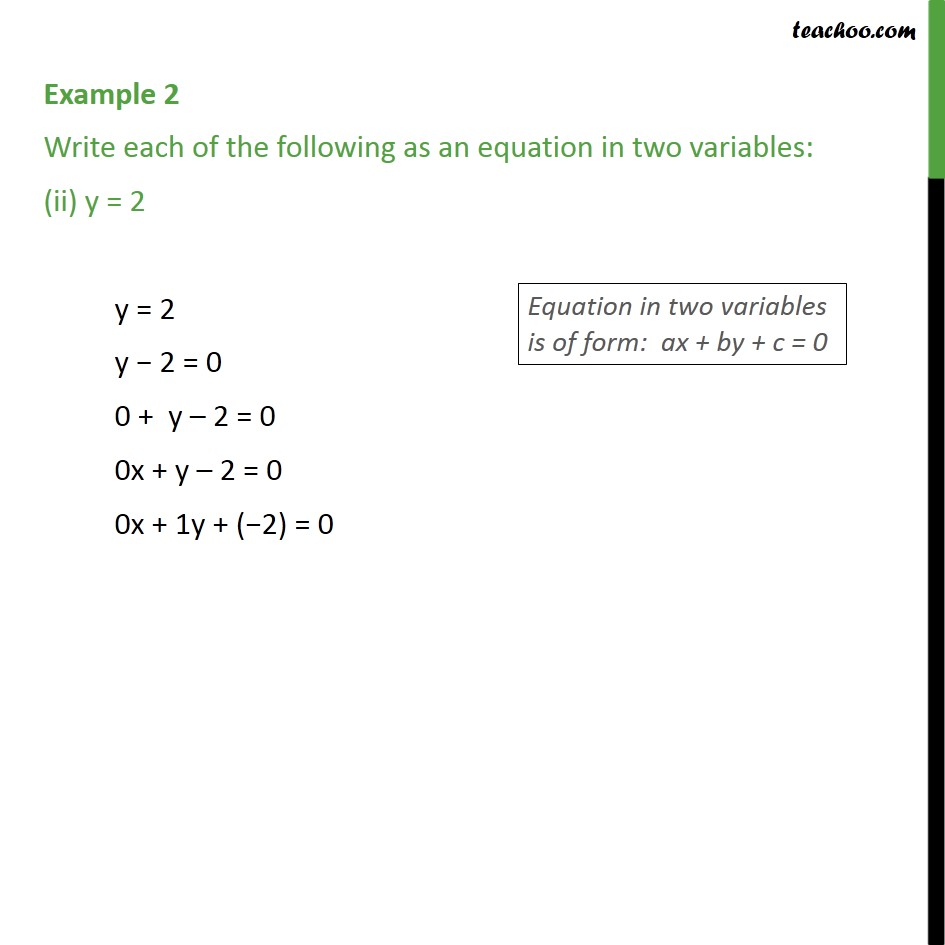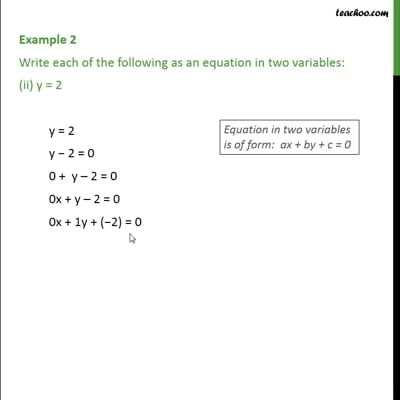Examples

Chapter 4 Class 9 Linear Equations in Two Variables
Serial order wiseThis video is only available for Teachoo black users

Maths Crash Course - Live lectures + all videos + Real time Doubt solving!

### Transcript

Example 2 Write each of the following as an equation in two variables: (ii) y = 2 y = 2 y 2 = 0 0 + y 2 = 0 0x + y 2 = 0 0x + 1y + ( 2) = 0